#### 期刊菜单

Seismic Response Analysis of Tongzi River Super Large Suspension Bridge
DOI: 10.12677/HJCE.2023.125078, PDF , HTML, XML, 下载: 82  浏览: 248  科研立项经费支持

Abstract: Long-span bridges are the hub of transportation and the lifeline of earthquake relief in mountainous areas. Once damaged in earthquake disasters, the consequences are very serious. Tongzi River Super Large Suspension Bridge is a long-span double-tower steel truss stiffened girder suspension bridge with a main span of 965 m, located in Tongzi County, Guizhou Province, with a large span, high main tower and complex structural stress. In order to evaluate the safety of the bridge under earthquake load, a space finite element model is established, and the response spectrum method is used to calculate and analyze the response of the Tongzi River Super Large Suspension Bridge under earthquakes of magnitude E1 and E2. The results show that under the action of dead weight + secondary dead load and transverse (or longitudinal) + vertical E1 and E2 earthquakes, the maximum tensile stress of the steel truss beam are 119.81 MPa, 129.12 MPa, 153.70 MPa and 173.53 MPa, respectively, and the maximum compressive stress are 87.12 MPa, 87.24 MPa, 79.09 MPa and 79.43 MPa, respectively, which are in the elastic working stage. Under the same working condition, the maximum tensile stress of the main tower under the action of corresponding E1 and E2 are 1.79 MPa, 2.09 MPa, 2.10 MPa and 2.39 MPa respectively, and the maximum compressive stress is 9.15 MPa, 8.72 MPa, 8.26 MPa and 7.84 MPa respectively, which meet the design requirements.

1. 引言

2. 工程概况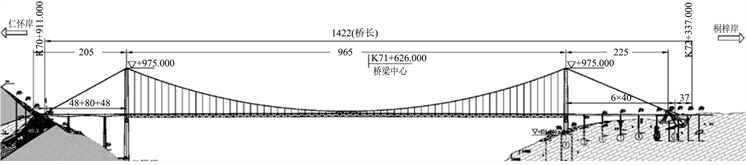Figure 1. General elevation of the project (m)Table 1. Main technical standards of the project

3. 建立有限元模型Table 2. Characteristic value of main materials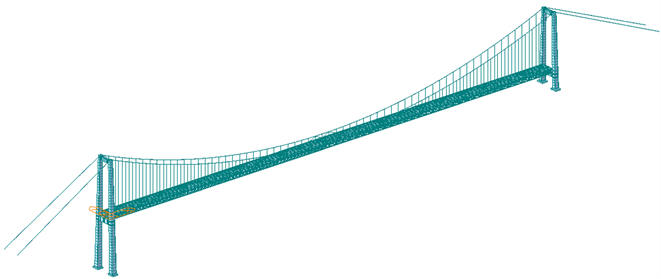Figure 2. Axial view of bridge finite element modelFigure 3. Partial axial view of truss and deck of bridge finite element model

4. 定义地震荷载组合

5. 输入反应谱数据Table 4. Seismic load related parameters

5.1. 反应谱函数定义

$S\left(T\right)=\left\{\begin{array}{ll}{S}_{\mathrm{max}}\left(0.6T/{T}_{0}+0.4\right)\hfill & T\le {T}_{0}\hfill \\ {S}_{\mathrm{max}}\hfill & {T}_{0} (1)

${S}_{\mathrm{max}}=2.5{C}_{i}{C}_{s}{C}_{d}A$ (2)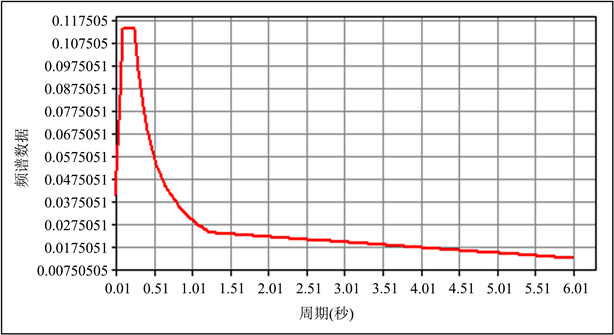Figure 4. E1 seismic response spectrum curve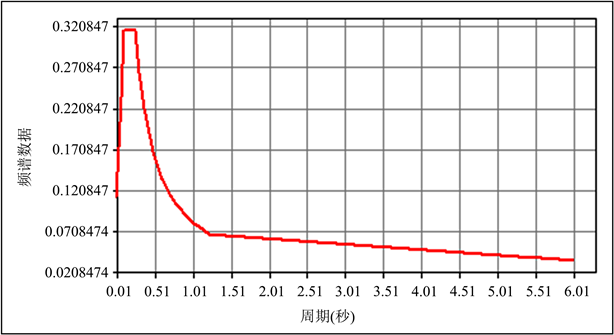Figure 5. E2 seismic response spectrum curve

5.2. 特征值定义

5.3. 反应谱荷载工况

CQC法的表达式如式(3)所示。

$F=\sqrt{\underset{i=1}{\overset{n}{\sum }}\underset{j=1}{\overset{n}{\sum }}{r}_{ij}{S}_{i}{S}_{j}}$ (3)

$F=\sqrt{\underset{i=1}{\overset{n}{\sum }}{S}_{i}^{2}}$ (4)

6. E1、E2地震作用下弹性反应谱计算结果及分析

6.1. 振型及频率Table 5. Calculation results of partial vibration mode period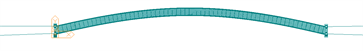(a) 第一阶振型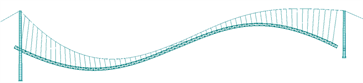(b) 第二阶振型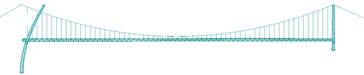(c) 第三阶振型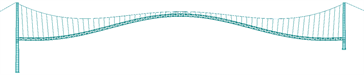(d) 第四阶振型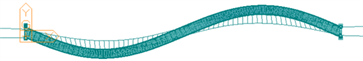(e) 第五阶振型(f) 第六阶振型

Figure 6. Shape diagram of the first six vibration modes of the bridge

6.2. 结构应力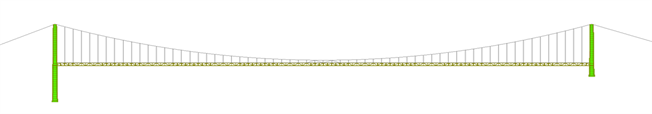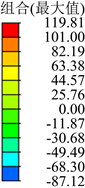(a) 整桥应力云图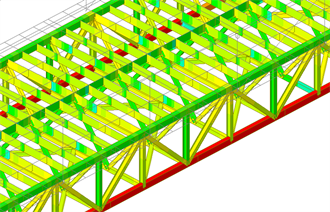(b) 拉应力最大处(跨中)云图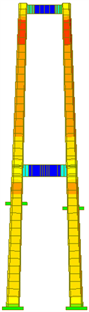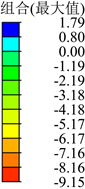(c) 混凝土桥塔应力云图

Figure 7. Stress cloud diagram under working condition 1 (MPa)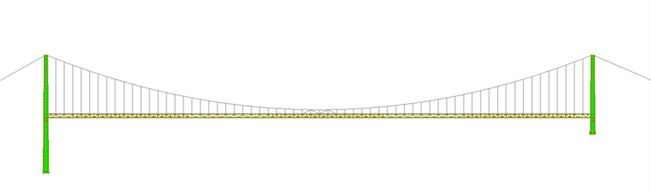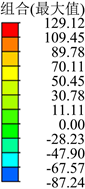(a) 整桥应力云图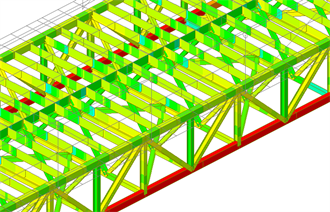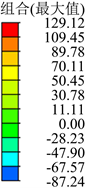(b) 拉应力最大处(跨中)云图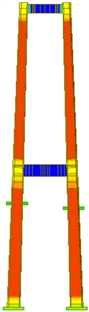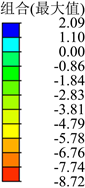(c) 混凝土桥塔应力云图

Figure 8. Stress cloud diagram under working condition 2 (MPa)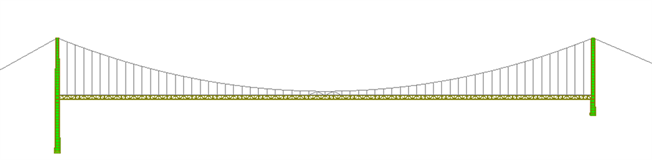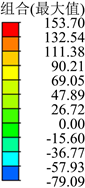(a) 整桥应力云图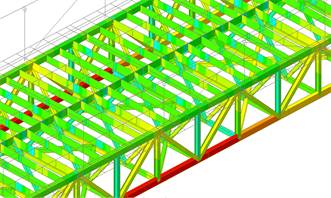(b) 拉应力最大处(跨中)云图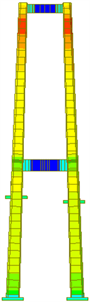(c) 混凝土桥塔应力云图

Figure 9. Stress cloud diagram under working condition 3 (MPa)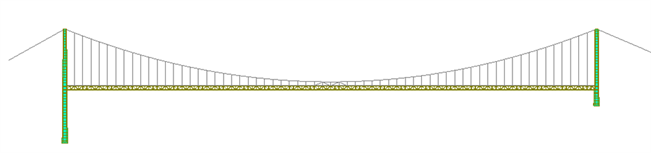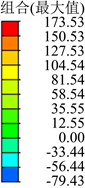(a) 整桥应力云图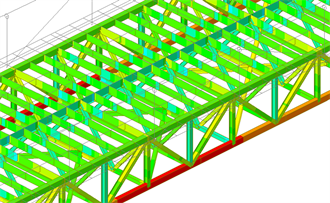(b) 拉应力最大处(跨中)云图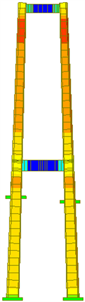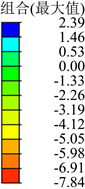(c) 混凝土桥塔应力云图

Figure 10. Stress cloud diagram under working condition 4 (MPa)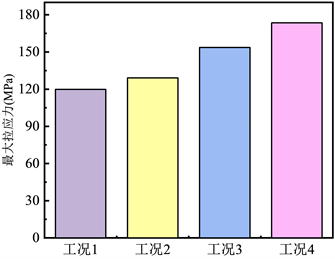Figure 11. Comparison diagram of maximum tensile stress (MPa)Table 6. The maximum tensile and compressive stress of the component under working condition 1 (MPa)Table 7. The maximum tensile and compressive stress of the component under working condition 2 (MPa)Table 8. The maximum tensile and compressive stress of the component under working condition 3 (MPa)Table 9. The maximum tensile and compressive stress of the component under working condition 4 (MPa)

7. 结论

NOTES

*通讯作者。

  任远航. 大跨度混合梁斜拉桥抗震分析研究[D]: [硕士学位论文]. 长沙: 中南大学, 2014.  李帅, 张凡, 颜晓伟, 等. 近断层地震动合成方法及其对超大跨斜拉桥地震响应影响[J]. 中国公路学报, 2017, 30(2): 86-97+106.  王晓伟, 叶爱君, 沈星, 等. 大跨度边墩横向减震体系的地震易损性分析[J]. 同济大学学报(自然科学版), 2016, 44(3): 333-340.  Sgambi, L., Garavaglia, E., Basso, N., et al. (2014) Monte Carlo Simulation for Seismic Analysis of a Long Span Suspension Bridge. Engineering Structures, 78, 100-111. https://doi.org/10.1016/j.engstruct.2014.08.051  Apaydin, N.M., Bas, S. and Harmandar, E. (2016) Response of the Fatih Sultan Mehmet Suspension Bridge under Spatially Varying Multi-Point Earthquake Excitations. Soil Dynamics and Earthquake Engineering, 84, 44-54. https://doi.org/10.1016/j.soildyn.2016.01.018  Adanur, S., Altunisik, A.C., Soyluk, K., et al. (2016) Multiple-Support Seismic Response of Bosporus Suspension Bridge for Various Random Vibration Methods. Case Studies in Structural Engineering, 5, 54-67. https://doi.org/10.1016/j.csse.2016.04.001  Gimsing, N.J. and Georgakis, C.T. (2012) Cable-Supported Bridges: Concept and Design. Wiley, Chichester. https://doi.org/10.1002/9781119978237  权新蕊, 宋松科, 刘伟. 近活动断裂带大跨悬索桥抗震性能研究[J]. 世界桥梁, 2021, 49(6): 57-63.  张新军, 张超. 大跨度悬索桥合理抗震结构体系研究[J]. 世界桥梁, 2017, 45(1): 39-44.  邓育林, 贾贤盛. 大跨度悬索桥地震反应中高阶振型的影响分析[J]. 工程抗震与加固改造, 2008(2): 24-28+81.  罗俊杰, 温留汉·黑沙. 结构构件阻尼比对大跨度悬索桥地震响应的影响[J]. 广州大学学报(自然科学版), 2016, 15(2): 16-20.  中华人民共和国交通运输部. 公路桥梁抗震设计规范: JTG/T 2231-01-2020[S]. 北京: 人民交通出版社, 2020.  中国地震局. 中国地震动参数区划图: GB18306-2015[S]. 北京: 中国标准出版社, 2015.  姚德隆. 基于Midas/civil的公路桥梁抗震安全性分析[D]: [硕士学位论文]. 衡阳: 南华大学, 2019.